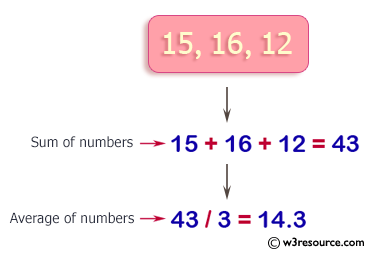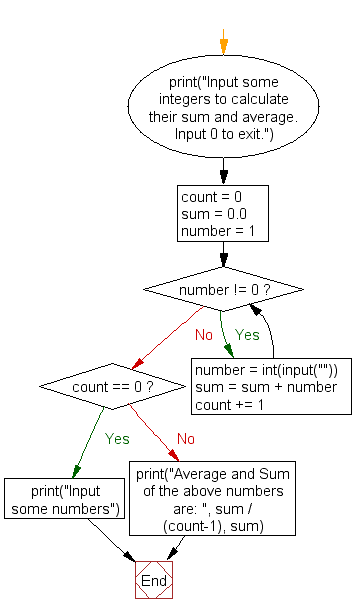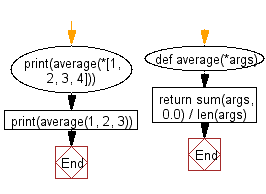﻿ Python Exercise: Calculate the sum and average of n integer numbers - w3resource

# Python Exercise: Calculate the sum and average of n integer numbers

## Python Conditional: Exercise - 42 with Solution

Write a Python program to calculate the sum and average of n integer numbers (input from the user). Input 0 to finish.

Pictorial Presentation:Sample Solution-1:

Python Code:

``````# Display a prompt asking the user to input integers to calculate their sum and average,
# specifying that entering 0 will exit the program
print("Input some integers to calculate their sum and average. Input 0 to exit.")

# Initialize variables for count, sum, and the first number to 1
count = 0
sum = 0.0
number = 1

# Iterate until the user inputs 0
while number != 0:
# Prompt the user to input a number and convert it to an integer
number = int(input(""))

# Add the entered number to the sum
sum = sum + number

# Increment the count of entered numbers
count += 1

# Check if no numbers were entered
if count == 0:
print("Input some numbers")
else:
# Calculate and display the average and sum of the entered numbers
print("Average and Sum of the above numbers are: ", sum / (count-1), sum)
```
```

Sample Output:

```Input some integers to calculate their sum and average. Input 0 to exit
.
15
16
12
0
Average and Sum of the above numbers are:  14.333333333333334 43.0
```

Flowchart :Sample Solution-2:

Calculates the average of two or more numbers.

Use sum() to sum all of the args provided, divide by len().

Python Code:

``````# Define a function 'average' that takes in a variable number of arguments using *args
def average(*args):
# Calculate the sum of the arguments using the built-in 'sum' function, starting from 0.0 as the initial value
# Divide the sum by the number of arguments using 'len(args)' to compute the average
return sum(args, 0.0) / len(args)

# Print the average of the elements within the list [1, 2, 3, 4] by unpacking it with '*'
print(average(*[1, 2, 3, 4]))

# Print the average of the numbers 1, 2, and 3 by passing them directly as arguments to the 'average' function
print(average(1, 2, 3))
```
```

Sample Output:

```2.5
2.0
```

Flowchart :Python Code Editor:

Have another way to solve this solution? Contribute your code (and comments) through Disqus.

What is the difficulty level of this exercise?

Test your Programming skills with w3resource's quiz.

﻿